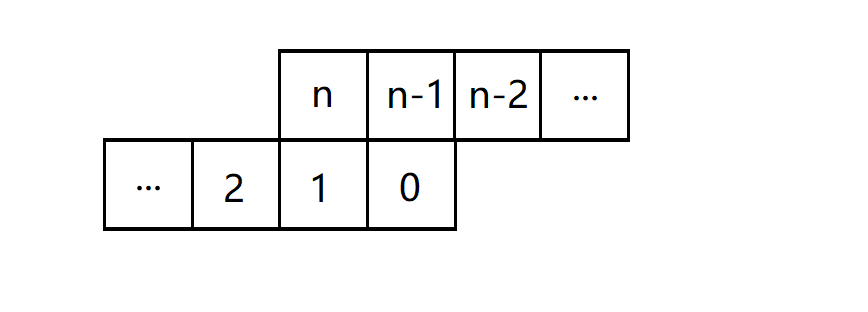# 「luogu 1896」 互不侵犯 - 轮廓线 dp

## 题目描述

$N\le 9,K\le N\times N$

## 题解$f_{i,j,k,t}$ 表示 第 $i$ 行 第 $j$ 列 放了 $k$ 个棋子 状态是 $t$ 时有多少种方案

#include <cstdio>
#include <cstring>
typedef long long ll;

const int MAXS=1025;
const int MAXK=82;

int N,K,cur;
ll res,f[MAXS][MAXK];

inline bool check(int i,int j,int s){
if (i!=1 && j!=1 && (s&(1<<N))) return 0;
if (i!=1 && (s&(1<<N-1))) return 0;
if (i!=1 && j!=N && (s&(1<<N-2))) return 0;
if (j!=1 && (s&1)) return 0;
return 1;
}

int main(){
scanf("%d%d",&N,&K);
f=1;
for (int i=1;i<=N;++i)
for (int j=1;j<=N;++j){
cur^=1;
memset(f[cur],0,sizeof(f[cur]));
for (int s=0;s<(1<<N+1);++s){
int now=(s&((1<<N)-1))<<1;
for (int p=0;p<=K;++p){
f[cur][now][p]+=f[cur^1][s][p];
if (p<K && check(i,j,s)) f[cur][now^1][p+1]+=f[cur^1][s][p];
}
}
}
for (int i=0;i<(1<<N+1);++i) res+=f[cur][i][K];
printf("%lld\n",res);
return 0;
}
posted @ 2018-09-24 15:51 xay5421 阅读(...) 评论(...) 编辑 收藏